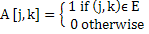# GATE | GATE-CS-2003 | Question 70

Let G (V, E) be a directed graph with n vertices. A path from vi to vj in G is sequence of vertices (vi, vi+1, ……., vj) such that (vk, vk+1) ∈ E for all k in i through j – 1. A simple path is a path in which no vertex appears more than once.
Let A be an n x n array initialized as follow

``

Consider the following algorithm.

```for i = 1 to n
for j = 1 to n
for k = 1 to n
A [j , k] = max (A[j, k] (A[j, i] + A [i, k]); ```

Which of the following statements is necessarily true for all j and k after terminal of the above algorithm ?
(A) A[j, k] ≤ n
(B) If A[j, k] ≥ n – 1, then G has a Hamiltonian cycle
(C) If there exists a path from j to k, A[j, k] contains the longest path lens from j to k
(D) If there exists a path from j to k, every simple path from j to k contain most A[j, k] edges

Explanation:

```In the original input matrix,  A[j , k] is 1 if there
is an edge from j to k, else 0.

Below expression is important to note:

A[j , k] = max(A[j, k] (A[j, i] + A [i, k]);

This expression puts the count of maximum edges on a path from
j to k.  In this expression, we consider every vertex k that can
become an intermediate vertex and can give longer path.```

My Personal Notes arrow_drop_up
Article Tags :

Be the First to upvote.

Please write to us at contribute@geeksforgeeks.org to report any issue with the above content.